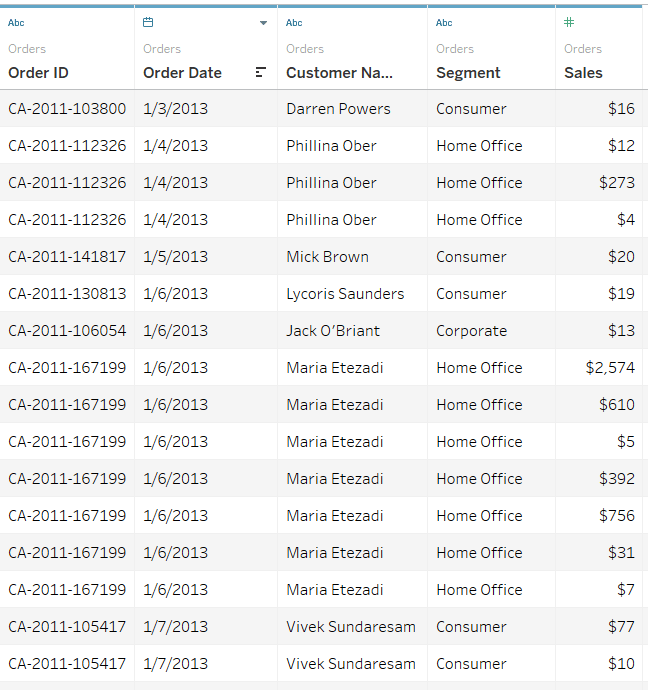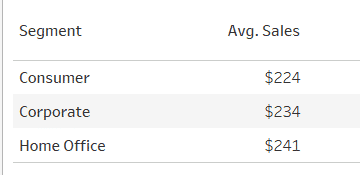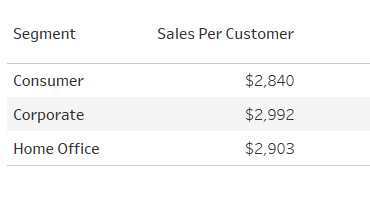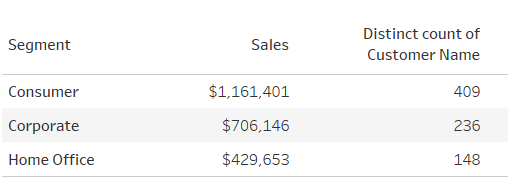# INCLUDE – LOD Deep Dive in Tableau

INCLUDE: As the name suggests, “INCLUDE” level of detail expression compute aggregations considering dimensions which are specified in the calculation and also take into consideration those dimensions which are present in the view.
“INCLUDE” level of detail expression is useful when the user wants to calculate values at the lower level of granularity and then aggregate and show the values at the higher level of granularity.

Example 1:
Requirement: Let’s consider the requirement is to compare the average customer sales across different product segments.
Data: The granularity of the data is “Order ID”Current view: Here in this view, if we directly use “Avg(Sales)” across “Segment” then it just shows the normal average value of sales taking into consideration all the respective “Order Id’s”.But, as the requirement is to show average customer sales across segments, in the view we are going to show only “Segment” but in calculations, we need to include “Customer Name” as well so that average customer sales can be calculated

Formula/Expression: AVG({INCLUDE [Customer Name] : SUM([Sales])})
Explanation:

1. In the above formula, we are including the lower level of dimension i.e. Customer Name to get the aggregate (sum) values of Sales at that level and then we are showing the average at higher level i.e. Segment. Below is the result of using the INCLUDE function:2. To understand how this, INCLUDE formula works, Let’s consider we use the “SUM(Sales)” and “Distinct count of Customer Name“ across Segments:
This represents the total sales across segments and the number of unique customers buying the under that segment.3. Now, if we divide the “SUM(Sales)” by “Distinct count of Customer Name“, then we will get the same value as shown in a).
4. This is what INCLUDE functions does in the backend of Tableau: AVG({INCLUDE [Customer Name] : SUM([Sales])}).
It takes the sum of total sales across the mentioned dimension i.e. Customer Name and then takes an average across the dimension present in the view i.e. Segment.

• in
• |
• October 23, 2018#### Author1

Subscribe
Notify of